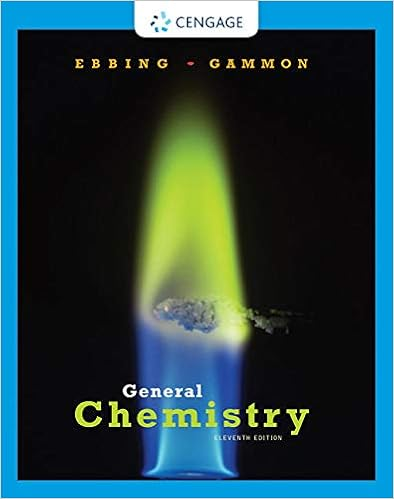Expt 34 Equilibrium Constant (1).pdf - Experiment 34 An...

• Lab Report
• 12
• 100% (2) 2 out of 2 people found this document helpful

This preview shows page 1 - 3 out of 12 pages.

We have textbook solutions for you!
The document you are viewing contains questions related to this textbook.The document you are viewing contains questions related to this textbook.
Chapter 13 / Exercise 13.89
General Chemistry
Ebbing/GammonExpert Verified
Experiment 34 An Equilibrium Constant To use a spectrophotometer to determine the equilibrium constant of a chemical system To use graphing techniques and data analysis to evaluate data To determine the equilibrium constant for a soluble equilibrium The following techniques are used in the Experimental Procedure: Experiment 34 371 Objectives Spectrophotometer: a laboratory instrument that measures the amount of light transmitted through a sample The nearly colorless iron(III) ion (left) forms an intensely colored complex (right) in the presence of the thiocyanate ion. Techniques Introduction A spectrophotometric method of analysis involves the interaction of electromag- netic (EM) radiation with matter. The most common regions of the EM spectrum used for analyses are the ultraviolet, visible, and the infrared regions. We are most familiar with the visible region of the spectrum, in which wavelengths range from 400 to 700 nm. The visible spectra of ions and molecules in solution arise from electronic tran- sitions within their respective structures. The greater the concentration of the absorb- ing ions/molecules in solution, the greater is the absorption of the visible EM radiation (and the greater the transmittance of the complementary radiation). The degree of absorbed radiation (or the intensity of the transmitted radiation) is mea- sured using an instrument called a spectrophotometer , which measures transmitted light intensities with a photosensitive detector at specific (but variable) visible wave- lengths (Figure 34.1, page 372). The wavelength where the absorbing ions or molecules has a maximum absorption of visible radiation is determined and set on the spectropho- tometer for the analysis. The visible light path through the spectrophotometer from the light source through the sample to the photosensitive detector is shown in Figure 34.2, page 372. Several factors control the amount of EM radiation (light energy) that a sample absorbs: Concentration of the absorbing substance Thickness of the sample containing the absorbing substance (determined by the width of the cuvet ) Probability of light absorption by the absorbing substance (called the molar absorptivity coef cient or extinction coef cient ) The Introduction to Dry Lab 3 discusses in more detail the interaction of electromagnetic radiation with atoms, ions, and molecules in terms of energy states, excited states, wavelengths, and spectra. Cuvet: a special piece of glassware to hold solutions for measurement in the spectrophotometer
We have textbook solutions for you!
The document you are viewing contains questions related to this textbook.The document you are viewing contains questions related to this textbook.
Chapter 13 / Exercise 13.89
General Chemistry
Ebbing/GammonExpert Verified
The ratio of the intensity of the transmitted light, I t , to that of the incident light, I 0 (Figure 34.3), is called the transmittance , T, of the EM radiation by the sample. This ratio, expressed as percent, is (34.1) Most spectrophotometers have a % T (percent transmittance of light) scale. Because it is linear, the % T scale is easy to read and interpolate. However, chemists often perform calculations based on the amount of light absorbed by the sample rather than the amount
•••